ENGR338 Lab 2021 Spring
Lab 1
Review Superposition, Thevenin's Equivalent Circuit, and LTSpice
Name: Sean Eaton
Email: smeaton@fortlewis.edu

Reviewing Superposition, Thevenin's Equivalent Circuit, and LTSpice

Introduction
In this lab we were tasked with reviewing various circuit analysis techniques. Superposition theory was used to analyze the circuit in Task 1 while Task 2 required us to find the Thevenin's Equivalent circuit. In addition to this, we had to verify our calculations with an LTSpice simulation. It was also required of us to implement the LTSpice simulation using only spice code.

Task 1: Review Superposition and Spice
For the following circuit use superposition theory to calculate all of the voltages and currents. Verify calculations using LTSpice and Spice code only.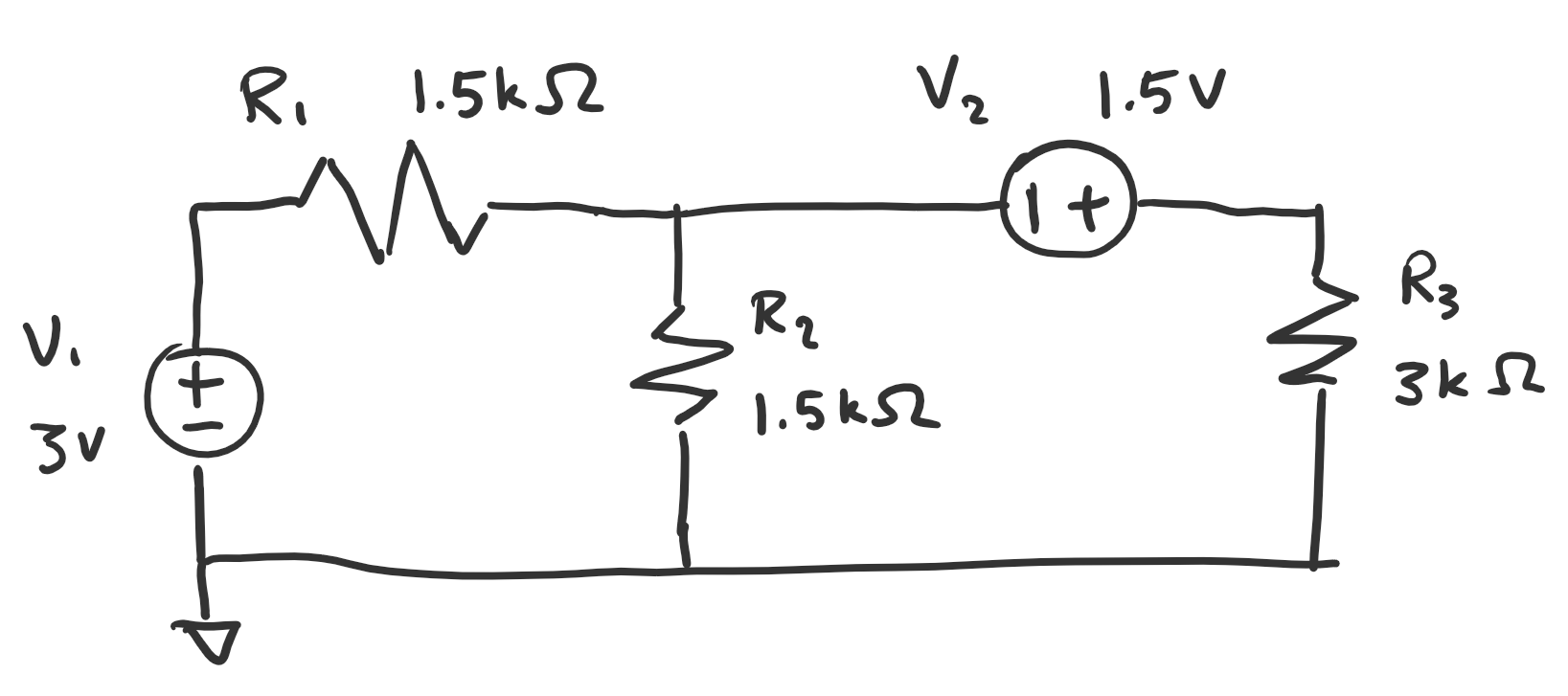Task 2: Review Thevenin's Equivalent Circuit and RC time delay
For the following circuit, we treated CL as the load and converted everything to the left side of it to its Thevenin's Equivalent circuit. The PULSE function was left to us to define. The only requirement was that it needed to show a nice time delay plot. We then had to calculate the time delay and compare it to the measured time delay.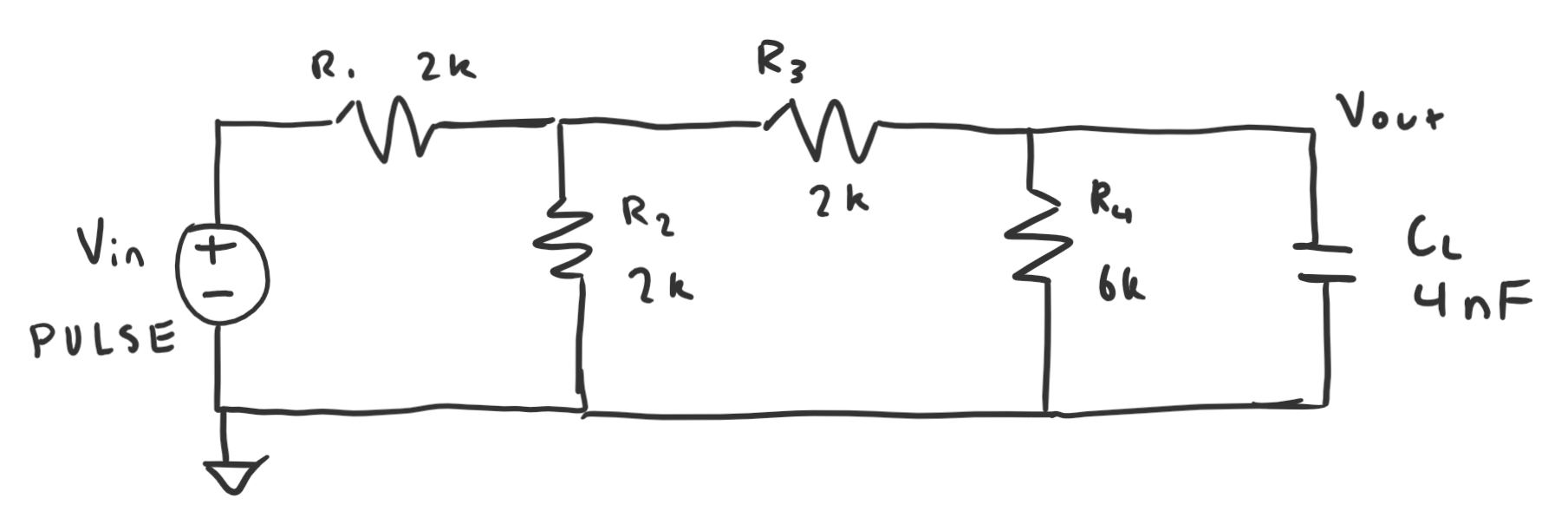Results
Task 1: Figure 3 shows the nodes I defined on the schematic.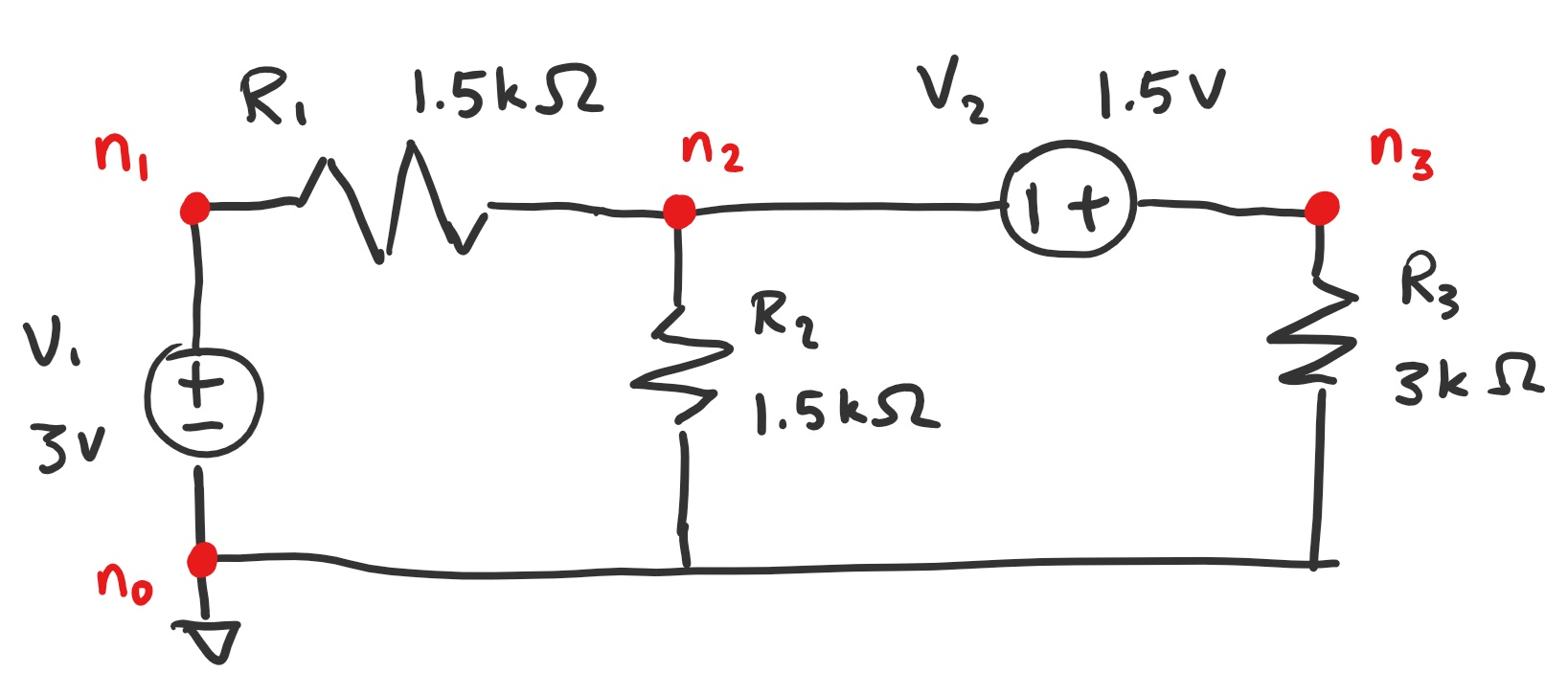Figure 3: Nodes labelled on the schematic.

For superposition we analyze the circuit using each voltage source on its own. Figure 4 below shows the calculations for voltage source 1 and voltage source 2 on their own. The voltages at each node was figured out as well as the currents running through each branch.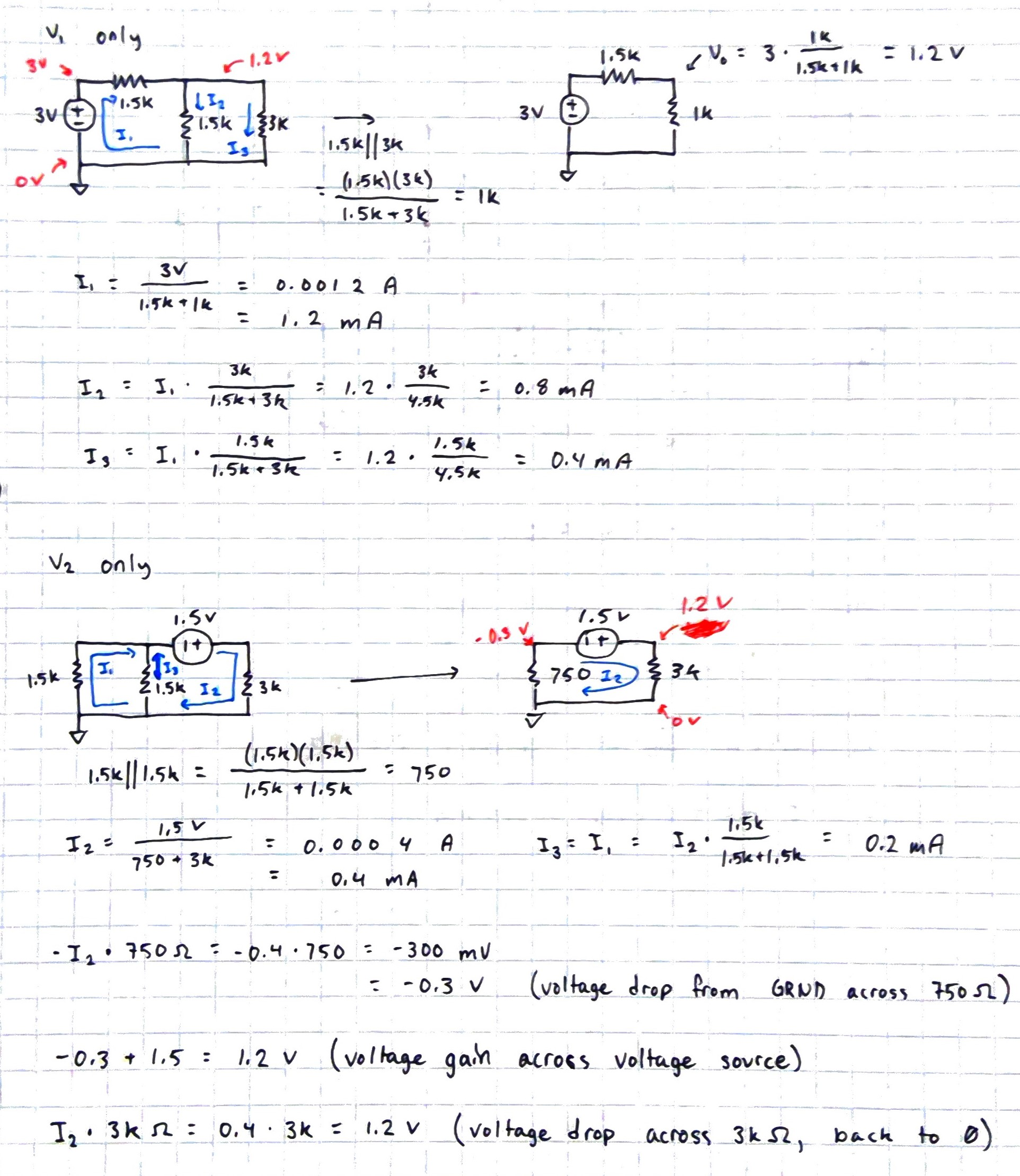Figure 4: Voltage and current calculations.

After finding the node voltages and currents for the circuits, the results are combined to get the node voltages and currents for the circuit containing both voltage sources. Figure 5 shows the results being put together to get the final results for the original circuit.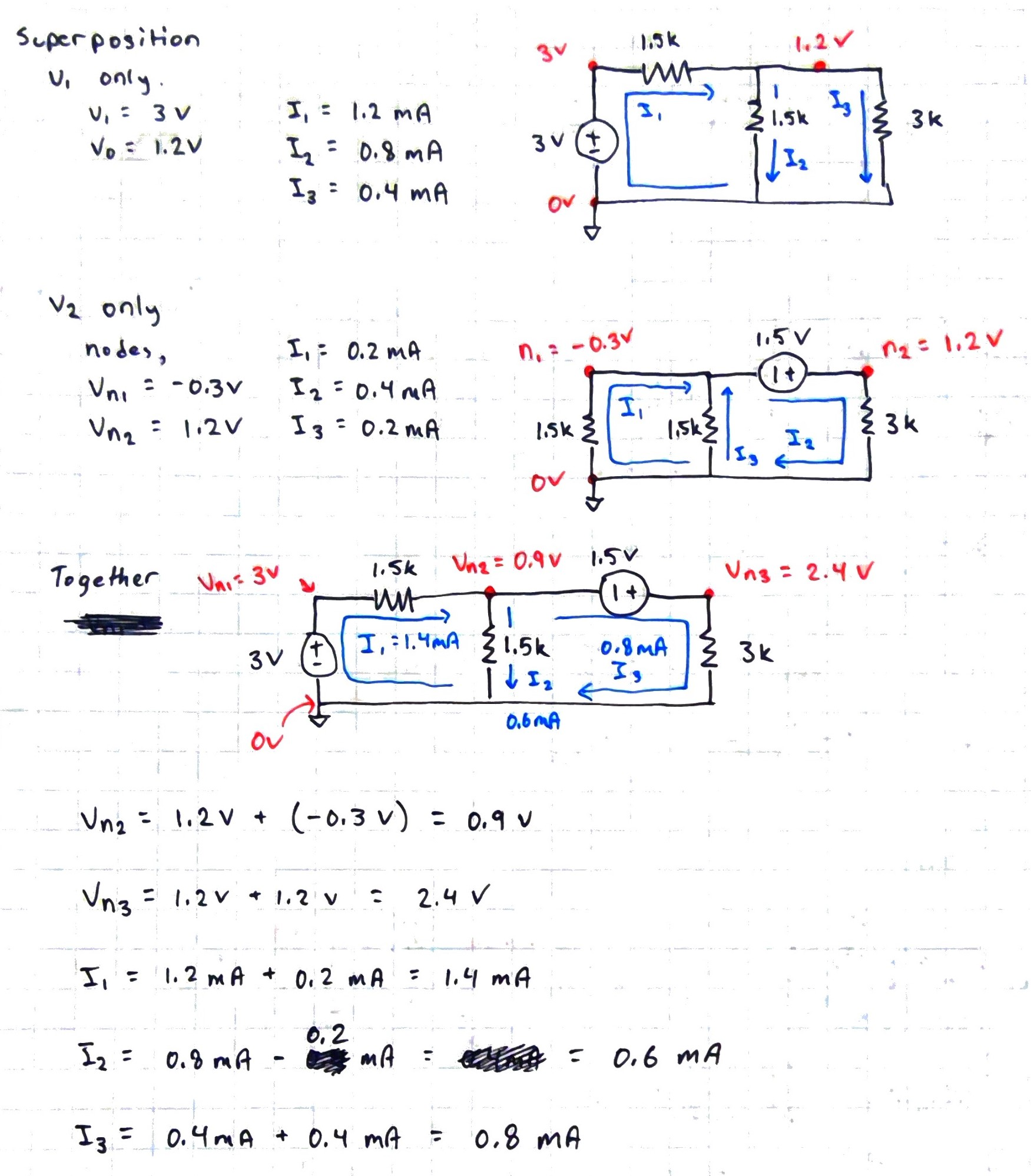Figure 5: Final superposition calculations.

This circuit was then designed in LTSpice using the following Spice code.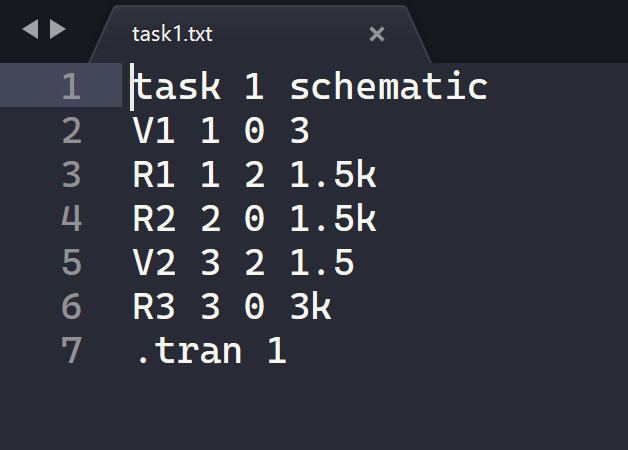Figure 6: Spice code for schematic 1.

In table 1, the calculated results and the simulated results for the node voltages will be compared.

 Nodes Calculated Simulated n0 0V 0V n1 3V 3V n2 0.9V 0.9V n3 2.4V 2.4V
Table 1: Calculated node voltages compared to simulated node voltages.

The figure below shows the plotted simulated results for the node voltages.Figure 7: Plotted node voltages for nodes 1, 2, and 3.

In table 2, the calculated results and the simulated results for the current branches are compared. They correspond to the resistor numbers.

 Currents Calculated Simulated I1 1.4 mA 1.4 mA I2 0.6 mA 0.6 mA I3 0.8 mA 0.8 mA
Table 2: Calculated currents compared to simulated currents.

In Figure 8 the simulated results were plotted in LTSpice.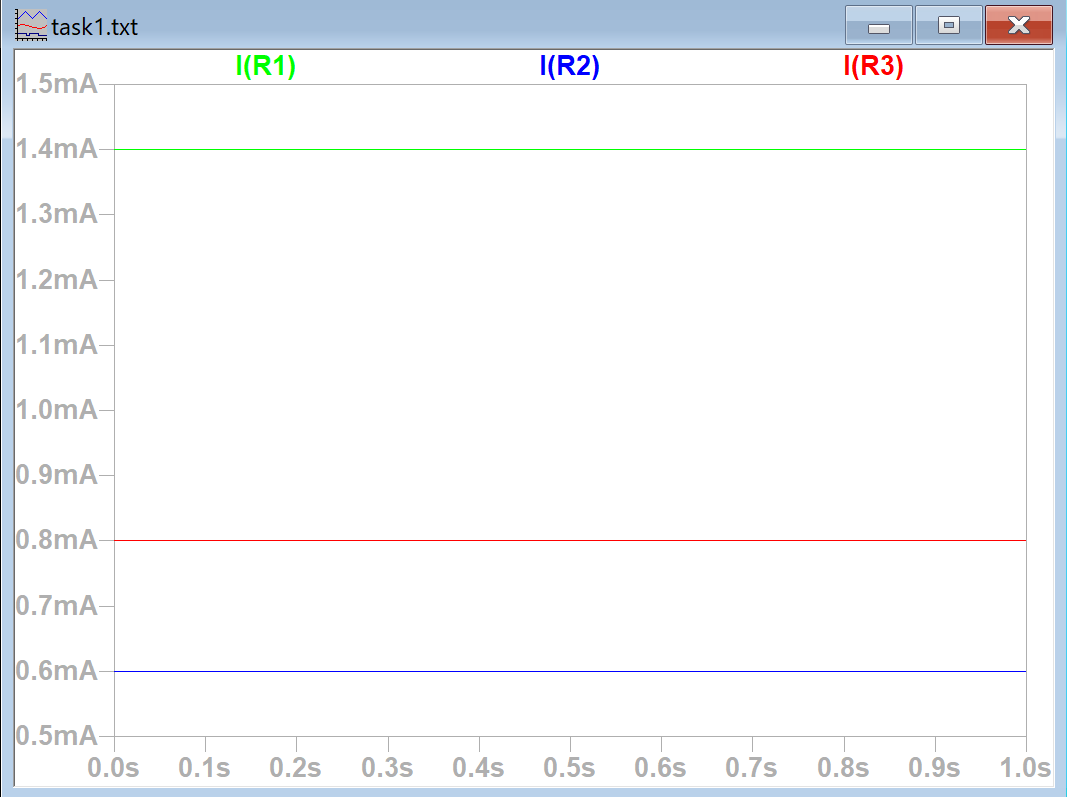Figure 8: Plotted currents for resistors 1, 2, and 3.

For task 2 the Thevenin's equivalent circuit and time delay was worked out before defining the PULSE function. Figures 9 and 10 show the calculations for the Thevenin's equivalent circuit and the time delay.Figure 9: Calculations for finding the Thevenin's equivalent resistance.

The crossed out circuit above in figure 9 had a mistake with the 2k ohm and 6k ohm resistors and was crossed out. In the end RTH was found to be 2k ohms. Some beginning work to find the equivalent voltage is shown on the bottom as well.Figure 10: Calculations for finding Thevenin's equivalent voltage and the time delay of the circuit.

The Thevenin's equivalent voltage was found to be one third of the input voltage of the original circuit. For the simulation I decided to implement the Thevenin's equivalent circuit instead of the original circuit. The calculated time delay was found to be 5.6 microseconds. The simulated time delay was measured to be 5.8 microseconds as shown below in Figure 11.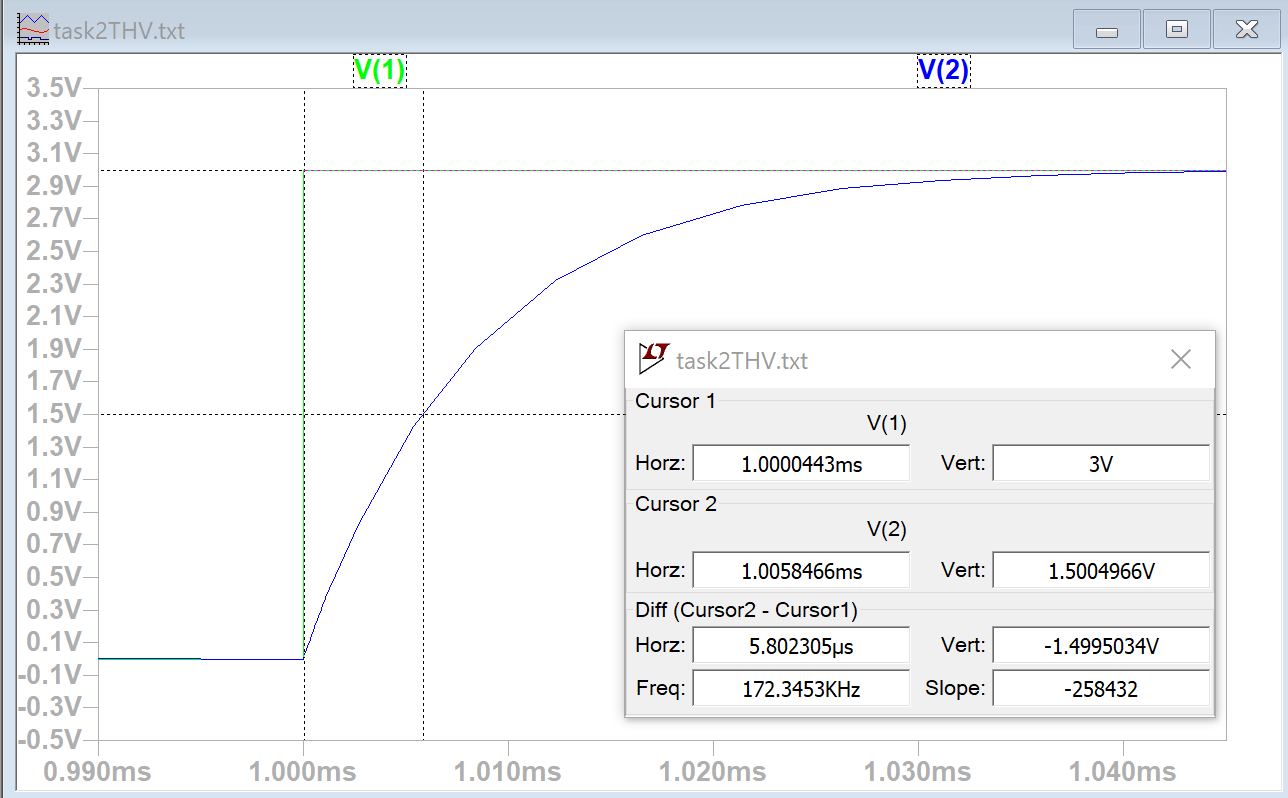Figure 11: Measuring time delay between ETH and Vout in the Thevenin's equivalent circuit.

The spice code for task 2's Thevenin's equivalent circuit is shown below in Figure 12.Figure 12: Schematic 2's Thevenin's equivalent circuit spice code.

Discussion

This lab was a good refresher on superposition and finding the Thevenin's equivalent circuit as well as using spice code in LTSpice which I hadn't used very often.
In task 1 the simulation results were not initially correct because my spice code defined the nodes connecting the second voltage source in the wrong order. It was helpful to learn more about spice code and how the nodes should be listed in the direction of the voltage drop and not how I would normally think about the direction of the nodes.
In task 2 I decided to simulate the Thevenin's equivalent circuit because it was simpler than the original schematic although I did simulate the original circuit. Since ETH equals one third of the original circuit's voltage input the plotting between Vin and Vout also looked better on the Thevenin's equivalent voltage compared to the original circuit. The difference between the calculated and simulated time delay is most likely due to how it was measured in LTSpice. If the cursor could be set to exactly 1.5V the measured value should match the calculated value.